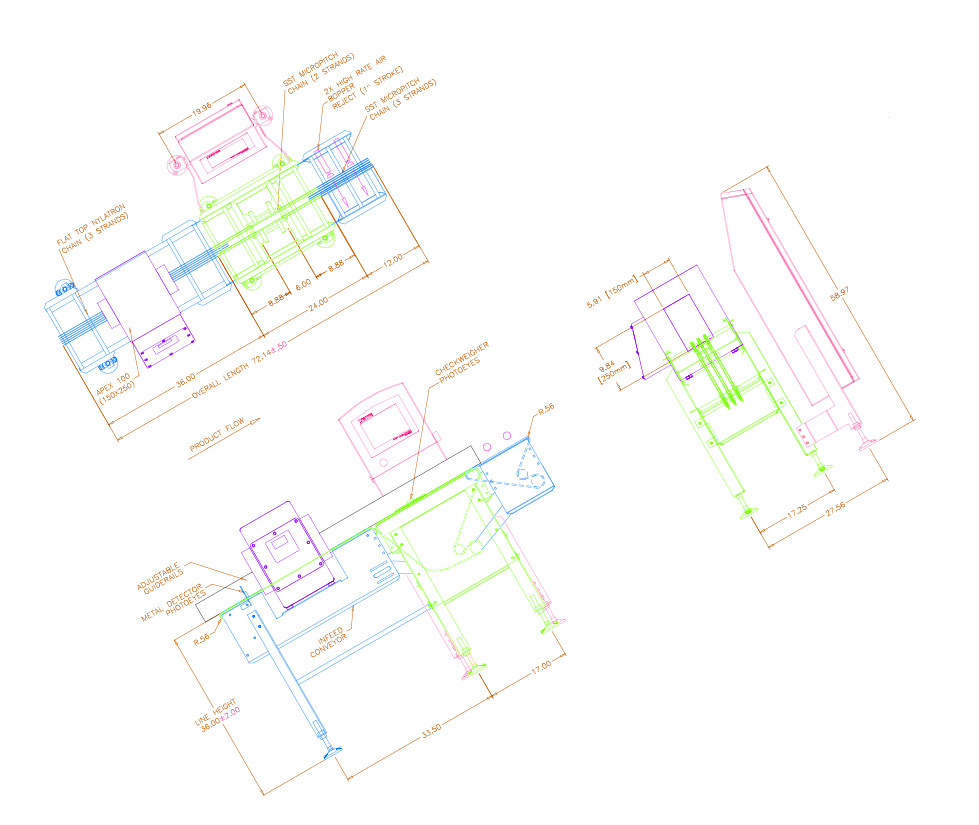## Sergey Sorvenkov

December 06, 2018

In addition to 3D objects, you can use ODA Publish SDK to create interactive documents that contain 2D CAD objects published from .dwg, .dgn, and BIM files. This article summarizes the Publish SDK classes used to publish drawings that contain 2D CAD objects.

The OdCADDefinition class is a repository for a CAD object exported to .pdf. The object is specified by the setDatabase function. The object is published (using its extents) and scaled according to the specified output canvas size specified by the setMediaSize function. The remaining functions of this class are similar to the PdfExport settings.

``````void setDatabase(OdDbBaseDatabase *database);
void setLayoutName(const OdString& name);

//The whole drawing will be published (using its extents) and scaled to fit the specified output media size
void setMediaSize(const Page::PaperUnits units, const double width, const double height);

void setEmbededTrueTypeFonts(bool state);
void setTrueTypeFontAsGeometry(bool state);
void setSHXTextAsGeometry(bool state);
void setTextSearchable(bool state);

void setGeomDPI(OdUInt32 dpi);
void setBWImagesDPI(OdUInt32 dpi);
void setColorImagesDPI(OdUInt32 dpi);
``````

The OdCADReference class is the PDF page display of a CAD document, which is an object stored in an OdCADDefinition. For each representation of a CAD object (OdCADDefinition), there can be several different maps of it.

``````void setCADDefinition(OdCADDefinitionPtr cad_definition);
void setBorder(bool state);
void setBorderColor(ODCOLORREF color);
void setBorderWidth(const OdUInt16 width);
void setClipBoundary(const Page::PaperUnits units, const OdGePoint2dArray& clipBoundary);
void setTransform(const OdGeMatrix2d& xfm);
void setScale(double scale);
void setTranslation(const OdGeVector2d& vec);
``````

#### Examples

To add a 2D CAD object to a PDF page of a document, there are two functions in the OdPage class.

``````void addCADReference(const OdCADReferencePtr& CADreference, const OdRect& location);
``````

This function adds a 2D CAD object to the page. The size and position of the object are determined by the rectangular area specified by the location parameter. In this case, it is possible to specify only one type of transformation: rotation (setRotation). When you rotate an object, it is automatically scaled so that its dimensions do not exceed the limits of the specified area. Other transformation functions (setTransform, setScale, setTranslation) with this method for adding an object to the page have no effect.

``````void addCADReference(const OdCADReferencePtr& CADreference);
``````

This function also adds a 2D CAD object to the page. The size and position of the object are determined by the transformation matrix specified in the OdCADReference object itself. The transformation matrix can be specified by the setTransform function or by a combination of the setScale, setRotation and setTranslation functions.

Below is an example of various 2D CAD objects(.prc; .dwg; BIM):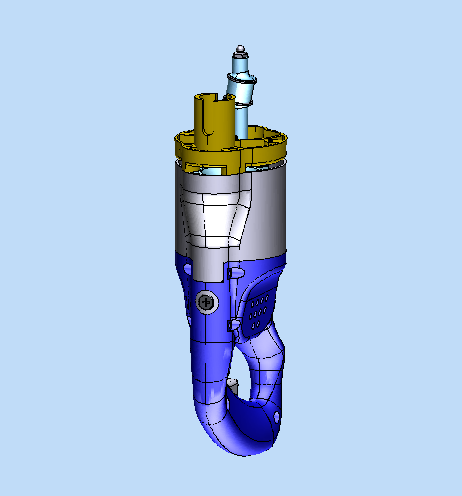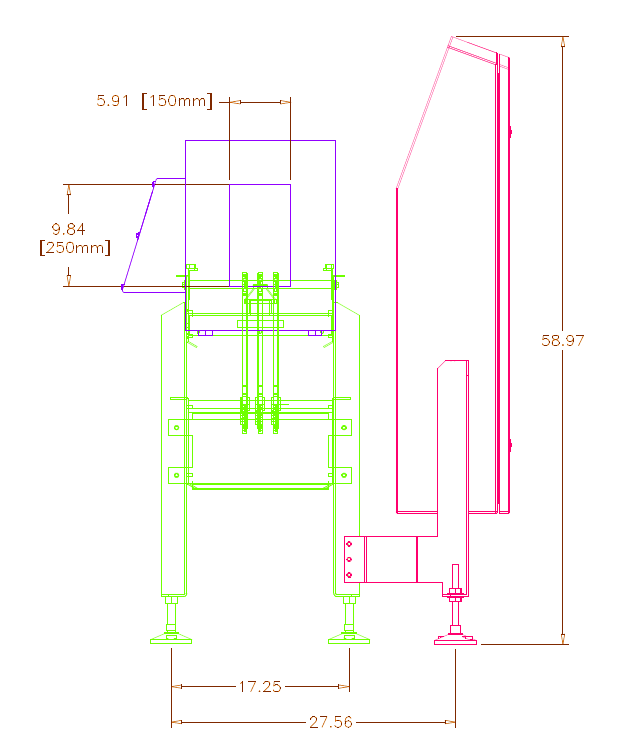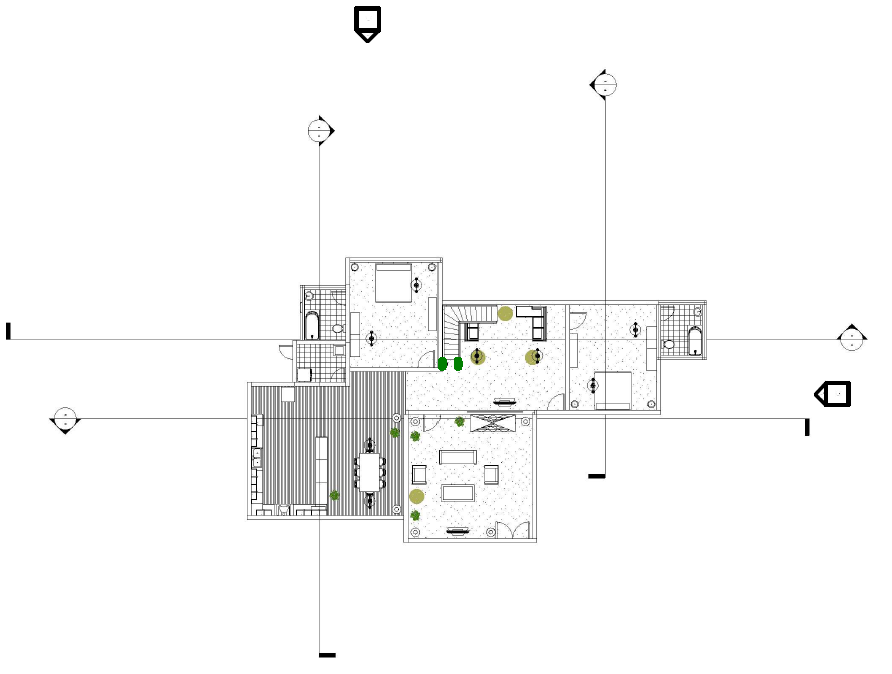Below is sample code for adding a 2D CAD object to a PDF document page. In this example, a CAD object is added to the page and rotated 45 degrees.

``````OdCADDefinitionPtr pCADDef = OdCADDefinition::createObject();

``````

The result of this code is shown next.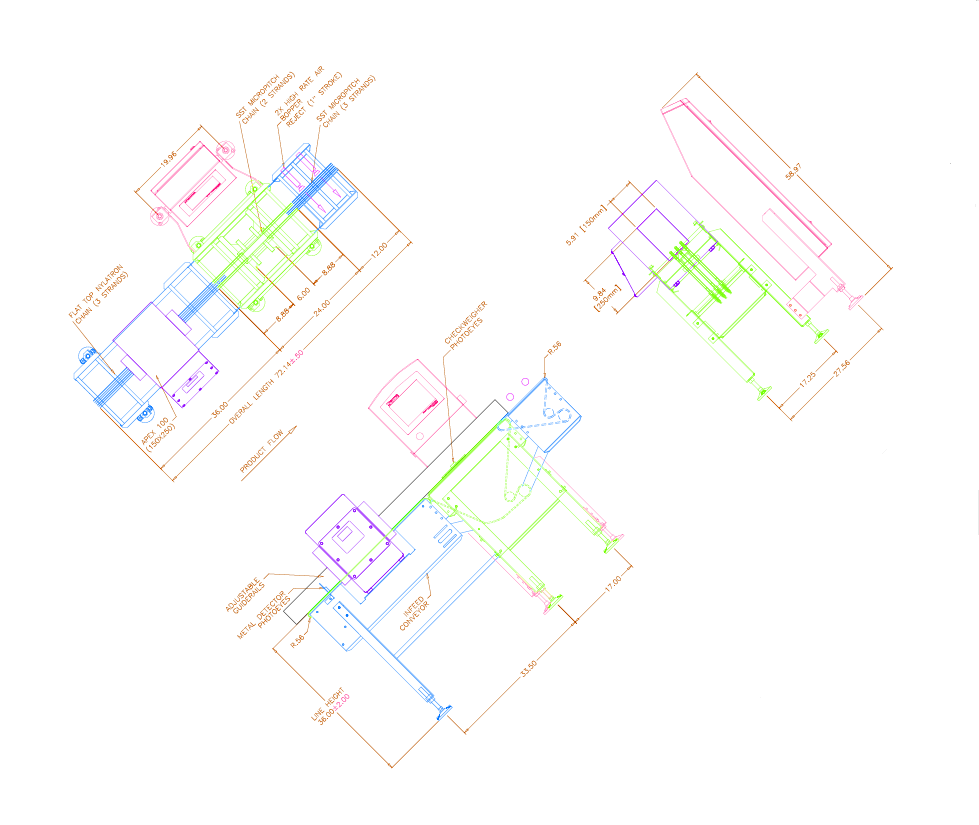To display only part of a drawing on the page, use the setClipBoundary function of the OdCADReference object. This function sets a polygonal area on the canvas (MediaSize) of a CAD object. Below is an example.

``````OdCADReferencePtr pCADRef3 = OdCADReference::createObject();
OdGePoint2dArray clip_box;
clip_box.append(OdGePoint2d(360, 100));
clip_box.append(OdGePoint2d(360, 350));
clip_box.append(OdGePoint2d(550, 350));
clip_box.append(OdGePoint2d(550, 100));
``````

The result of this code is shown below. The left side shows an example of using the OdTable object.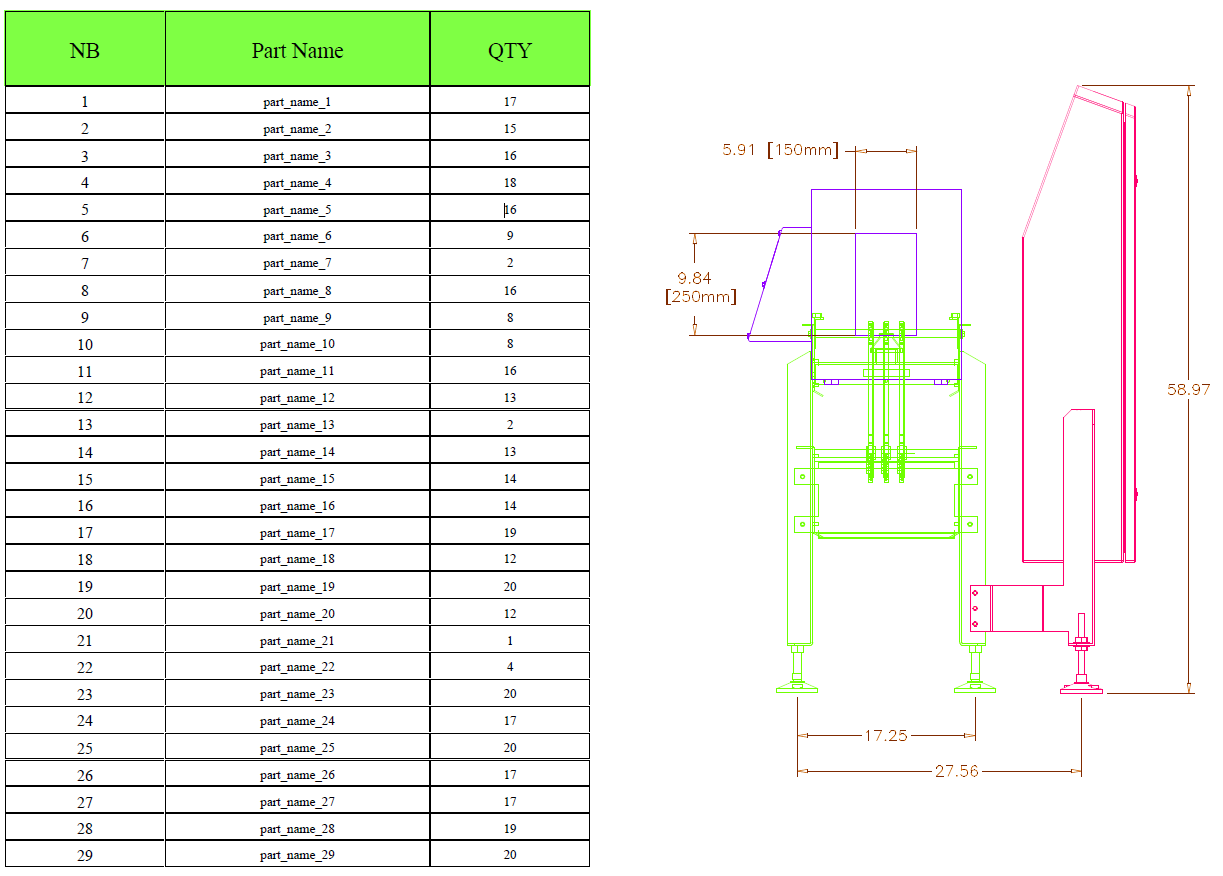The following code demonstrates how to add a CAD object using a transformation matrix.

``````OdCADReferencePtr pCADRef4 = OdCADReference::createObject();
OdGeMatrix2d transform;
transform.setCoordSystem(OdGePoint2d(350, 50), OdGeVector2d::kXAxis, OdGeVector2d::kYAxis);
transform *= OdGeMatrix2d::scaling(0.035);
transform *= OdGeMatrix2d::rotation(OdaPI/6.0);